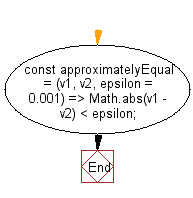# JavaScript: Check if two given numbers are approximately equal to each other

## JavaScript fundamental (ES6 Syntax): Exercise-256 with Solution

Write a JavaScript program to check if two given numbers are approximately equal to each other.

• Use Math.abs() to compare the absolute difference of the two values to epsilon.
• Omit the third argument, epsilon, to use a default value of 0.001.

Sample Solution:

JavaScript Code:

``````//#Source https://bit.ly/2neWfJ2
const approximatelyEqual = (v1, v2, epsilon = 0.001) => Math.abs(v1 - v2) < epsilon;
console.log(approximatelyEqual(Math.PI / 2.0, 1.5708));
```
```

Sample Output:

```133
100
```

Flowchart:Live Demo:

See the Pen javascript-basic-exercise-256-1 by w3resource (@w3resource) on CodePen.

Improve this sample solution and post your code through Disqus

What is the difficulty level of this exercise?

Test your Programming skills with w3resource's quiz.

﻿

## JavaScript: Tips of the Day

How to check whether a string contains a substring in JavaScript?

ECMAScript 6 introduced String.prototype.includes:

```const string = "foo";
const substring = "oo";

console.log(string.includes(substring));
```

includes doesn't have Internet Explorer support, though. In ECMAScript 5 or older environments, use String.prototype.indexOf, which returns -1 when a substring cannot be found:

```var string = "foo";
var substring = "oo";

console.log(string.indexOf(substring) !== -1);
```

Ref: https://bit.ly/3fFFgZv